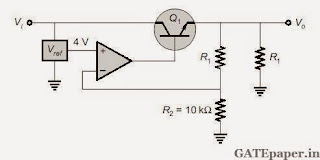GATE 2015 Question papers with complete Answer Key for all braches released by IIT Kanpur

GATE 2015 : Answer Key

Date / Day Answer Key
Session No. Time Paper Codes (For Answer Key)
31st January 2015
(Saturday)
1 9:00 Hrs - 12:00 Hrs
[FN Session]
AG, AR, BT, CH, CY, EC, EY, XE, XL
2 14:00 Hrs - 17:00 Hrs
[AN Session]
EC, ME,
1st February 2015
(Sunday)
3 9:00 Hrs - 12:00 Hrs
[FN Session]
EC, ME,
4 14:00 Hrs - 17:00 Hrs
[AN Session]
AE, GG, IN, MA, ME, MN, MT, PH, PI, TF
7th February 2015
(Saturday
)
5 9:00 Hrs - 12:00 Hrs
[FN Session]
CS, EE
6 14:00 Hrs - 17:00 Hrs
[AN Session]
CS, EE
8th February 2015
(Sunday)
7 9:00 Hrs - 12:00 Hrs
[FN Session]
CE, CS
8 14:00 Hrs - 17:00 Hrs
[AN Session]
CE

GATE 2015 ECE Digital Circuits (Digital Electronics) - Video Solutions

GATE 2015 ECE – Questions on Digital Circuits with Solutions
1. The circuit shown consists of JK flip flops, each with an active LOW asynchronous reset. The counter corresponding to this circuit is ___________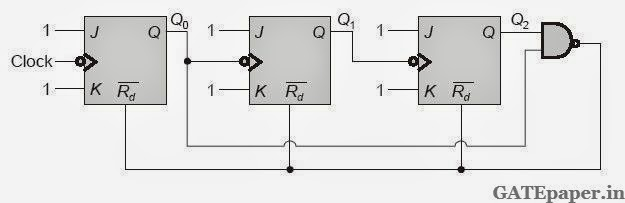a. Mod – 5 binary UP counter
b. Mod – 6 binary DOWN counter
c. Mod – 5 binary DOWN counter
d. Mod – 6 binary UP counter

2. In the circuit shown, D1, D2 and D3 are ideal and inputs E1, E2 and E3 are 0 volts for logic’0’ and 10 volts for logic ‘1’. What logic gate does the circuit represent?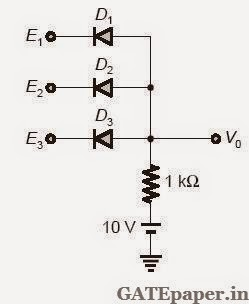a. 3 input OR gate
b. 3 input NOR gate
c. 3 input AND gate
d. 3 input XOR gate

3. A universal gate can implement any Boolean function by connecting sufficient number of them appropriately. Three gates are shown.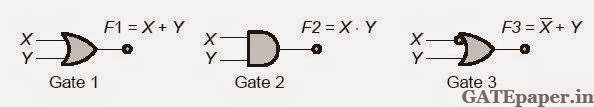Which one of the following statements is TRUE?
a. Gate 1 is universal gate
b. Gate 2 is universal gate
c. Gate 3 is universal gate
d. None of the gates shown is universal gate

4. A three bit pseudo random number generator is shown. Initially the value of output Y = Y2Y1Y0 is set to 111. The value of output Y after three clock cycles is _________a. 000
b. 001
c. 010
d. 100

5. An SR latch is implemented using TTL gates as shown in the figure. The set and reset pulse inputs are provided using the push button switches. It is observed that the circuit fails to work as desired. The SR latch can be made functional by changinga. NOR gates to NAND gates
b. Inverters to Buffers
c. NOR gates to NAND gates and inverters to buffers
d. 5 volts to ground

6. Consider a four bit Digital to Analog converter. The analog value corresponding to a digital signals of values 0000 and 0001 are 0 volts and 0.0625 volts respectively. The analog value (in volts) corresponding to the digital signal 1111 is ______________

7. In a 8085 microprocessor, the shift registers which store the result of an addition and the overflow bit are respectively
a. B and F
b. A and F
c. H and F
d. A and C

8. A 16 KB memory array is designed as a square with an aspect ratio of one (number of rows is equal to the number of columns). The minimum number of address lines needed for the row decoder is ______________

9. A 3 input majority gate is defined by the logic function M(a,b,c) = ab + bc + ca. Which one of the following gate is represented by the function M(M’(a,b,c),M(a,b,c’),c)?
a. 3 input NAND gate
b. 3 input XOR gate
c. 3 input NOR gate
d. 3 input XNOR gate

10. The Boolean expression F(x,y,z) = x’yz’+xy’z’+xyz’+xyz converted into canonical product of sum (POS) form is ___________11. All the logic gates shown in the figure have a propagation delay of 20 ns. Let A=C=0 and B=1 until time t = 0. At t = 0, all the inputs flip (i.e. A=C=1 and B=0) and remain in that state. For t>0, output z=1 for a duration (in 20 ns) of____________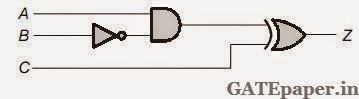12. A Mod-N counter using a synchronous binary UP counter with asynchronous clear input is shown in the figure. The value of N is_______________13. In the figure shown, the output Y is required to be Y = AB+C’D’. The gates G1 and G2 must be _____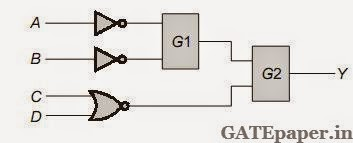a. NOR, OR
b. OR, NAND
c. NAND, OR
d. AND, NAND

14. In an 8085 microprocessor, which one of the following instructions changes the content of the accumulator?
a. MOV B,M
b. PCHL
c. RNZ
d. SBI BEH .

15. A function of Boolean variables X,Y and Z is expressed in terms of the min-terms as F(X,Y,Z) = ∑m(1,2,5,6,7). Which one of the product of sums given below is equal to the given function?16. The figure shows a binary counter with synchronous clear input. With the decoding logic shown, the counter works as a _______________a. Mod – 2 counter
b. Mod – 4 counter
c. Mod – 5 counter
d. Mod – 6 counter

17. Problem on Design of 1 to 8 De-multiplexer using two 2 to 4 decoders with extra logic gates.GATE 2015 ECE Analog Circuits - Video Solutions

GATE 2015 ECE – Questions on Analog Circuits with Answers
1. In the circuit shown, assume OP-AMP is ideal then the 3 dB cut off frequency (in Hz) is __________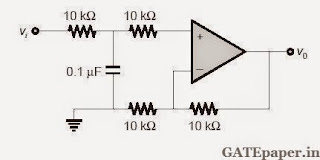2. Consider the bode plot shown in figure. Assume that all the poles and zeros are real valued. The value of fH – fL (in Hz) is _____________3. At very high frequencies, the peak output voltage Vo (in volts) is ______________4. In the circuit shown, assume that the OP-AMP is ideal. If the gain (Vo/Vin) is –12 , then the value of R (in kΩ) is _________________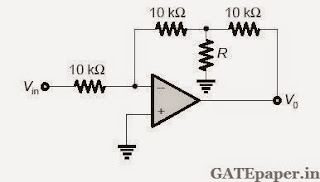5. Negative feedback in a closed loop control system DOES NOT
a. Reduce the overall gain
b. Reduce bandwidth
c. Improve disturbance rejection
d. Reduce sensitivity to parameter variation

6. In the circuit shown, assume that the OP-AMP is ideal. The bridge output voltage Vo (in mV) for δ = 0.05 is _______________7. The circuit shown in the figure has an ideal OP-AMP. The oscillation frequency and the condition to sustain the oscillations respectively are ________________8. In the circuit shown, I1 = 80 mA and I2 = 4 mA. Transistors T1 and T2 are identical. Assume that thermal voltage is 26 mV at 27oC. At 50oC, the value of voltage V12 = V1 – V2 (in mV) is _______________9. In the circuit shown, Vo = VOA for switch SW in position A and Vo = VOB for switch SW in position B. Assume that the OP-AMP is ideal. The value of VOB/VOA is ______________10. If the circuit shown has to function as a clamping circuit, then which one of the following conditions should be satisfied for the sinusoidal signal of period T?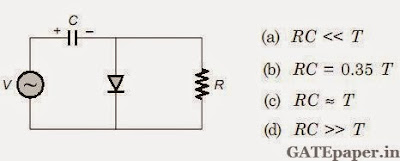11. In the bistable circuit shown, the ideal OP-AMP ahs saturation levels of ± 5 volts. The value of R1 (in kΩ) that gives a hysteresis width of 500 mV is _________________12. In the ac equivalent circuit shown, the two BJTs are biased in active region and have identical parameters with β>>1. The open circuit small signal voltage gain is approximately is _________________13. Assuming that the OP-AMP in the circuit shown is ideal, the output voltage Vo (inn volts) is __________